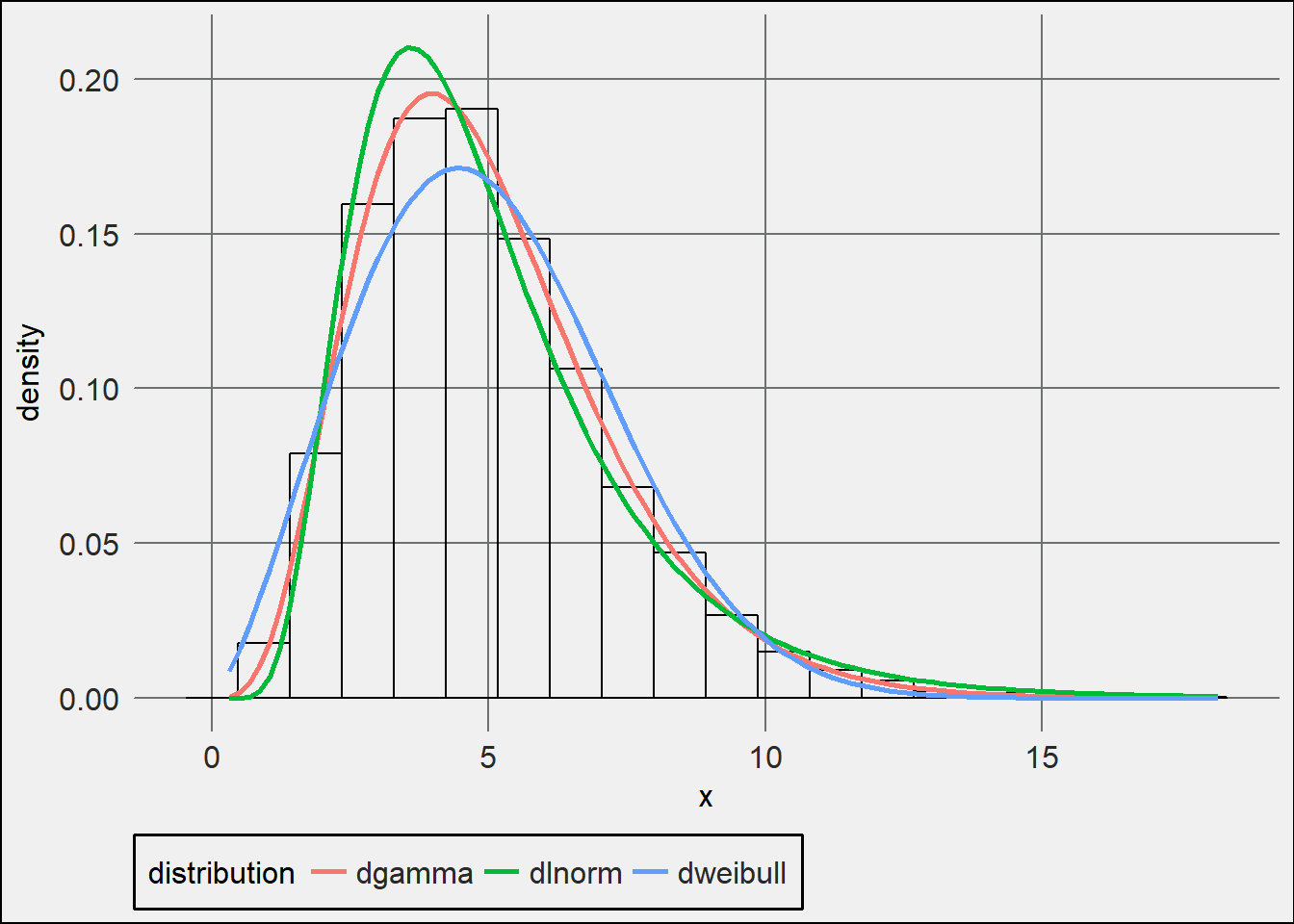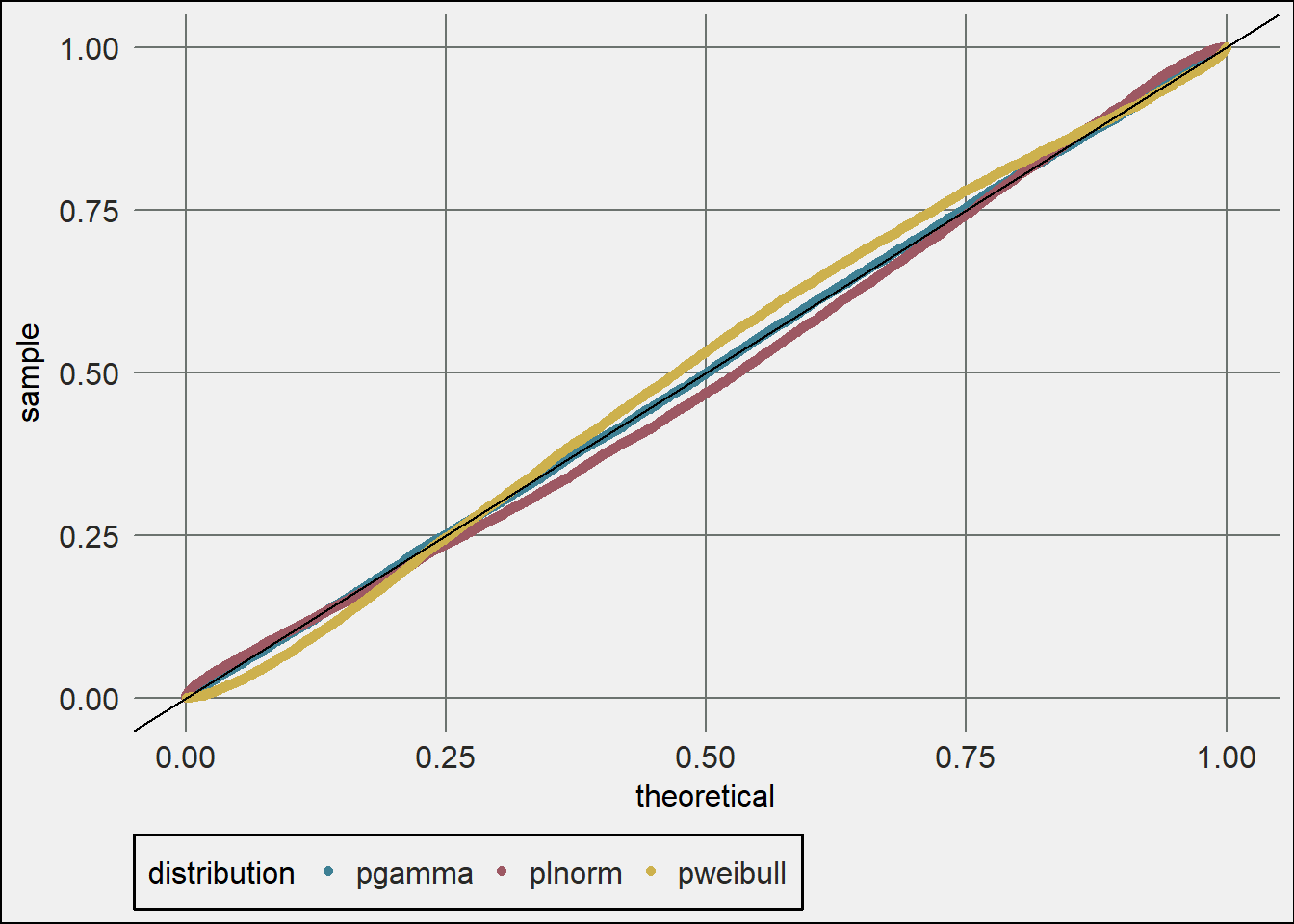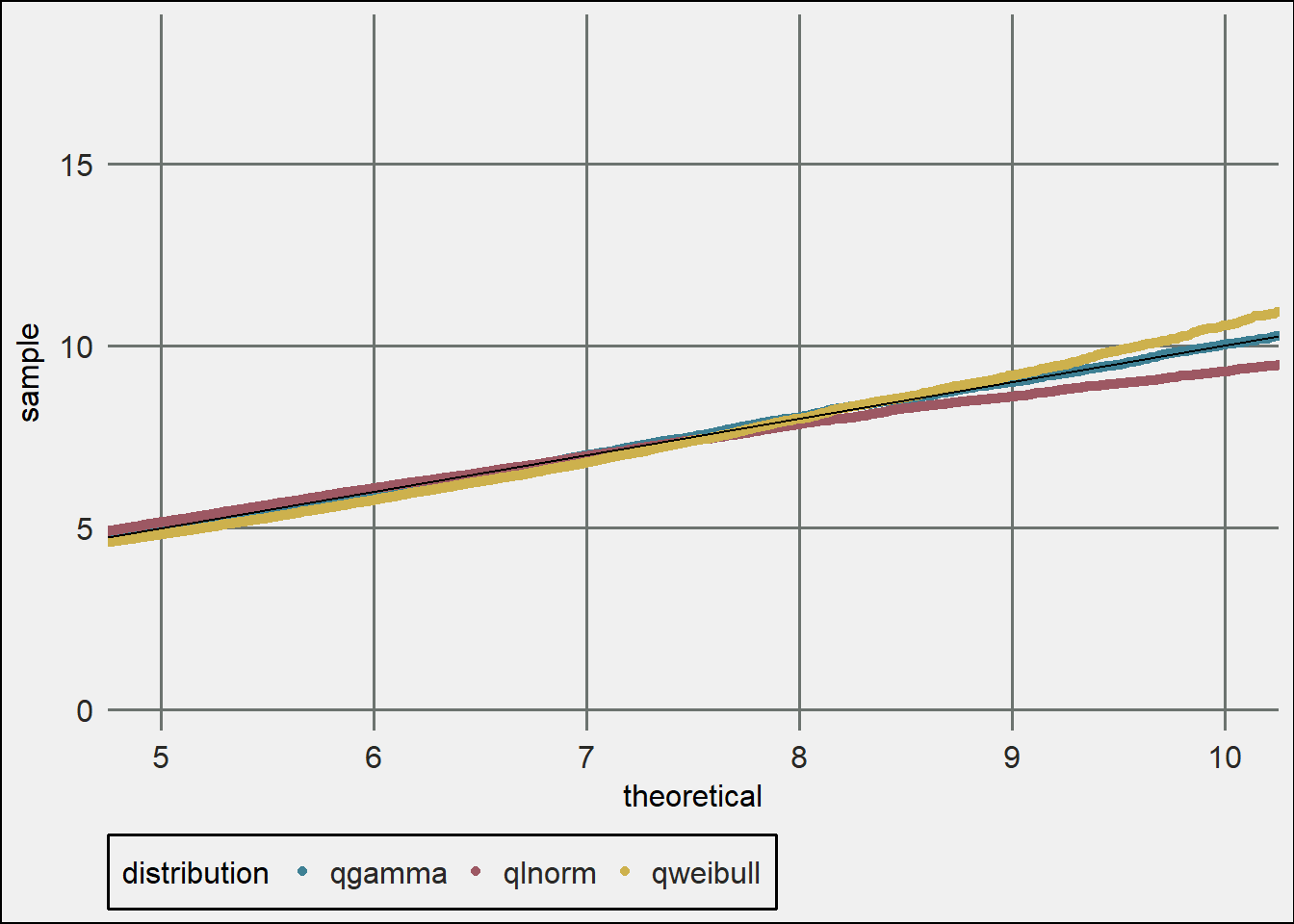#### fitur 0.5.25 Release

##### March 14, 2018
R fitur

• Fixed appearance of plots

• Added plot_density function for comparison pdfs of fitted functions

• Updated argument naming conventions

fitur on CRAN

## Density Diagnostic Plot

``````library(fitur)
library(ggplot2)
library(trstyles)
set.seed(37)
x <- rgamma(10000, 5)
dists <- c('gamma', 'lnorm', 'weibull')
fits <- lapply(dists, fit_univariate, x = x)
theme_set(theme_tr())
plot_density(x, fits, nbins = 20)``````## QQ-Plot

The output is a ggplot object so you can add colors, styling, etc.

``````plot_pp(x, fits) +
scale_color_tr()``````## PP-Plot

You can also zoom in on different areas.

``````plot_qq(x, fits) +
scale_color_tr() +
coord_cartesian(xlim = c(5,10))``````